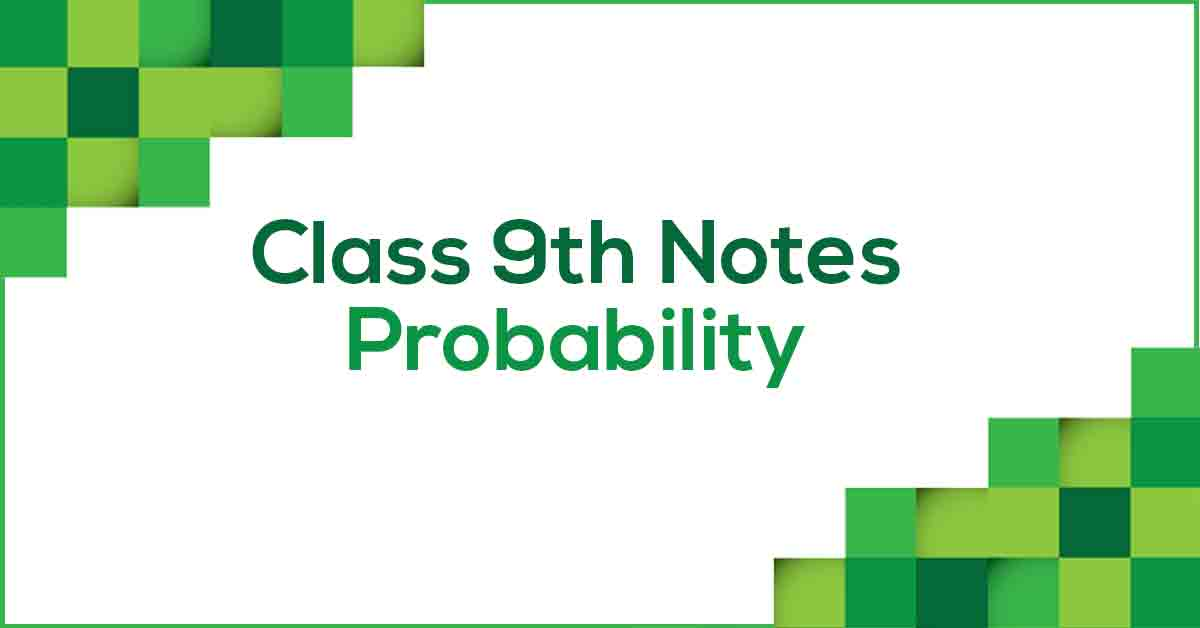Probability Class 9 NotesClass 9 Maths Chapter 15 Probability Notes - PDF Download

Probability Class 9 Notes are prepared strictly according to the NCERT Syllabus which helps to get rid of any confusion among children regarding the course content since CBSE keeps on updating the course every year. These notes are prepared by our panel of highly experienced teachers which not only reduces the pressure on the students but also offer them a simple way to study or revise the chapter. Probability Class 9 Notes would provide you with an effective preparation for your examinations

Understanding Probability.

Probability is the measure of the likeliness that an event will occur. Probability is quantified as a number between 0 and 1 (where 0 indicates impossibility and 1 indicates certainty). In mathematics, when next outcome of the experiment cannot be determined then we say it is a random experiment. A trial is an action which results in one or several outcomes, for example each toss of the coin and each throw of the die are called trials.

Learn more about trails, experiments and events in Probability Class 9 Notes pdf.